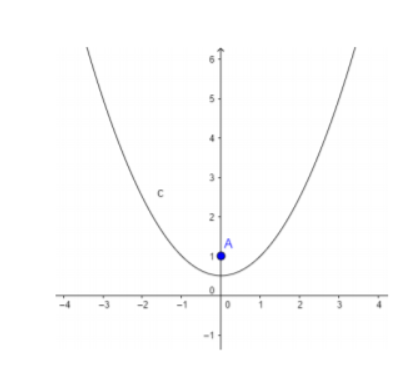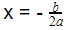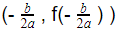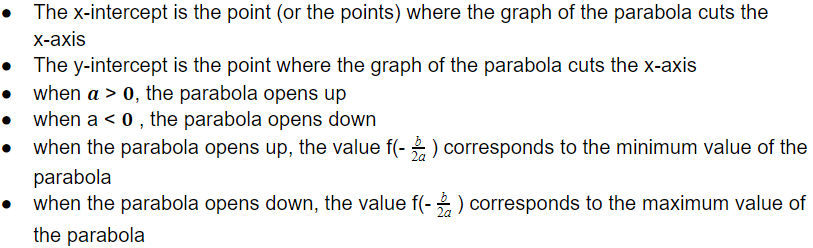# The Properties of Parabolas

 Would You Rather Listen to the Lesson?When algebra 2 students learn about quadratic equations and functions, they learn about the properties of parabolas. Thus, they become proficient in sketching any parabola, as well as in writing its properties.

Although students may sometimes find parabola lessons tedious, math teachers and homeschooling parents can make these lessons more inviting by employing a few cool teaching strategies. Read on to learn more.

## Strategies to Teach the Properties of ParabolasBefore jumping into the properties of parabolas, you can provide a brief overview of quadratic functions, as parabolas are the graphs of quadratic functions. Remind students that a quadratic function is a function of this form: f(x) = ax² + bx + c, where a is not equal to zero.

Also add that in f(x) = ax² + bx + c, ax² is a quadratic term, bx is a linear term, and c is a constant term. In quadratic functions, the highest degree term is of the second degree, as opposed to linear functions, where the highest degree term is of the first degree.

By now, students should be familiar with what a quadratic function looks like, so write a few functions on the whiteboard and ask students to determine which ones are quadratic and which ones aren’t. You can include the following functions:

• f(x) = 7x² – x – 1
• f(x) = 2x + 1
• f(x) = x²
• f(x) = – x² + 2x + 3

Provide a few minutes for students to reflect and then open space for discussion. How did students determine whether the given function is quadratic? In which functions is the highest degree term of the second and in which of the first degree?

### Graph of a Quadratic Function

Add that the graph of a quadratic function is always a vertical parabola. You can also draw such a parabola on the whiteboard as an example. This graph has a U shape, which is sometimes upside down.Remind students that there is a simple way in which they can check whether the parabola will have a U shape or it will be upside down. We can do this by observing the leading coefficient a – if it’s greater than 0, the parabola will be a U shape; if it’s less than zero, it will be upside down.

### Properties of Parabolas

Explain that each parabola has an axis of symmetry, which is the horizontal or vertical line that divides the parabola into two symmetrical parts. Add that mathematically, we represent the axis of symmetry in the following way:Then, explain that the vertex of the parabola is the point that intersects the axis of symmetry of the parabola. Mathematically, its coordinates are given as:Add that the vertex is the highest or lowest point on the graph for a parabola with a vertical axis of symmetry; whereas for a parabola with a horizontal axis of symmetry, the vertex is the furthermost left or right point on the graph.

Then, explain that there are several properties of parabolas, including:If you have the technical means in your classroom, you can also enrich your lesson on the properties of parabolas with multimedia materials, such as videos. Videos are especially useful to illustrate the different parabola drawings and their properties.

For instance, this video provides a simple explanation of what the axis of symmetry is and the vertex, and introduces the basic properties of parabolas when graphing a quadratic equation. This video also offers good practical examples of the properties of parabolas.

Finally, use this video to provide guidelines on how to graph quadratic functions, and then find the properties of the given parabolas. The video also contains practical examples that will guide students throughout the process.

## Activities to Practice the Properties of Parabolas

### Group Work

This activity will help students practice graphing quadratic functions, as well as writing the properties of parabolas. To use this activity in your classroom, the only thing you need to do is print out enough copies of this Assignment Worksheet (Members Only).

Divide students into groups of 3 and 4 and hand out the copies. Explain to students that the worksheet contains several exercises related to the properties of parabolas, such as finding the minimum and maximum value, x and y-intercepts, vertex, etc.

Students in each group work together to solve the exercises. Provide a few minutes for this. Then open space for discussion and reflection, asking each group to present their work in front of the class.

## Before You Leave…

If you like these strategies on teaching the properties of parabolas, and you’re looking for more algebra 2 resources, make sure to sign up for our emails to receive loads of free lessons and content!

In addition, you can sign up for a membership on MathTeacherCoach or head over to our blog, where you’ll find more math materials for kids of all ages. You’ll discover that with the resources we offer, teaching math has never been easier!